# Problem 58284. Easy Sequences 117: Fractional Part of Cube Roots

The fractional part function of a positive real number r, denoted as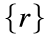, is defined as: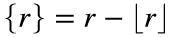, where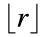, is the floor of r. Thus,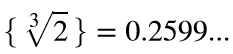,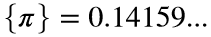and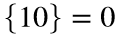.
Given a positive integer n, create the function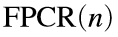, that evaluates the following summation:For example for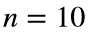:Please present the function output rounded-off to nearest 3 decimal places Therefore, for, the function should return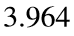.
---------------

### Solution Stats

60.0% Correct | 40.0% Incorrect
Last Solution submitted on Sep 11, 2023

### Community Treasure Hunt

Find the treasures in MATLAB Central and discover how the community can help you!

Start Hunting!